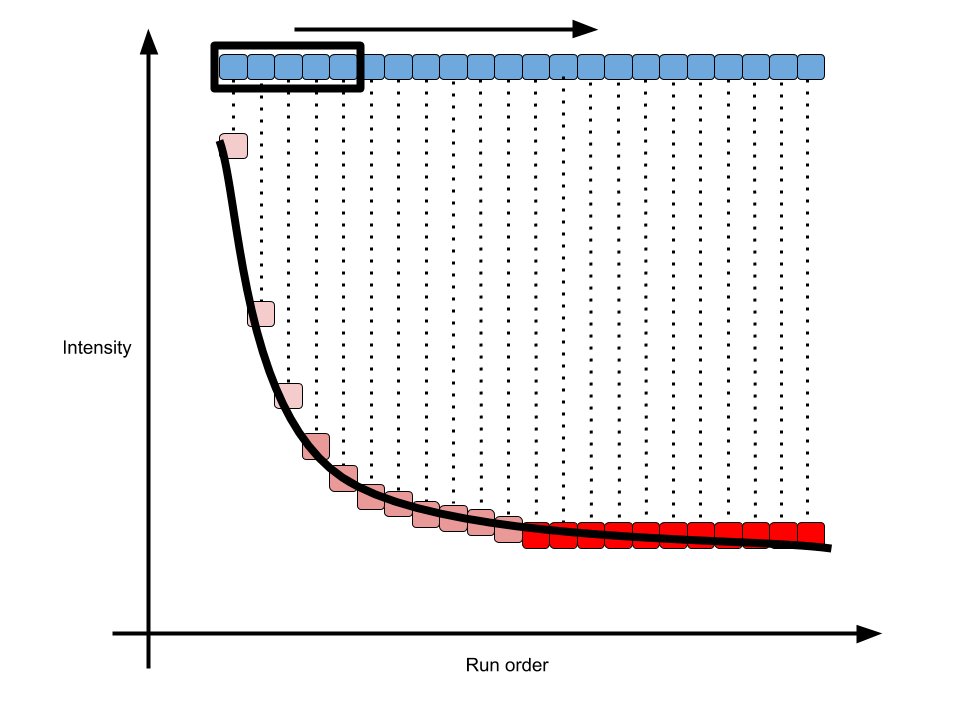# Pooled QC analysis In enviGCMS: GC/LC-MS Data Analysis for Environmental Science

knitr::opts_chunk$set( collapse = TRUE, comment = "#>" )  library(enviGCMS)  Pooled QC samples within injection sequences are very important to control the quality of untargeted analysis. I added some functions in enviGCMS package to visualize or analysis Pooled QC samples or other untargeted peaks profile. ## Peaks profile We could check sample-wise changes of peaks. plotpeak(list$data, lv = as.factor(list$group$sample_group))


Or peak-wise changes of samples.

plotpeak(t(list$data))  ## Density distribution For each sample, the peaks' density distribution will tell us if certain samples show large shift. data(list) plotden(list$data,list$group$sample_group,ylim = c(0,1))
# 1-d density for multiple samples


## Relative Log Abundance Ridge (RLAR) plots

Relative Log Abundance Ridge (RLAR) plots could also be used to show the intensity shift.

data(list)
plotridges(list$data, as.factor(list$group$sample_group)) # ridgeline density plot plotridge(t(list$data),indexy=c(1:10),xlab = 'Intensity',ylab = 'peaks')
plotdwtus(list)


## Run order effect analysis

For LC/GC-MS analysis, the run order will affect the intensity of single peak until the instrument is stable. The intensity will decrease/increase with initial run order and researcher need to evaluate how many samples are enough to eliminated run order effects. Here I introduced a pooled QC linear index to show such trends in the sequence.As shown in above figure, for one peak repeated analyzed in one sequence, the intensity would become stable in long term. In math, the slope of every 5 samples along the run order would become 0. Then we could define the percentage of stable peaks as pooled QC index. Such index would be a value between 0 and 1. The higher of such index, more peaks within the QC would be affected by run order effect. You could use such function to check the QC samples to see if run order effects would influence the samples at the beginning of sequences.

order <- 1:12
# n means how many points to build a linear regression model
n = 5
idx <- getpqsi(list\$data,order,n = n)
plot(idx~order[-(1:(n-1))],pch=19)


In this case, we could see at 5th sample, 30% peaks show correlation with the run order. However, ever since 6th sample, the run order effects could be ignore.

## Try the enviGCMS package in your browser

Any scripts or data that you put into this service are public.

enviGCMS documentation built on July 8, 2020, 5:52 p.m.